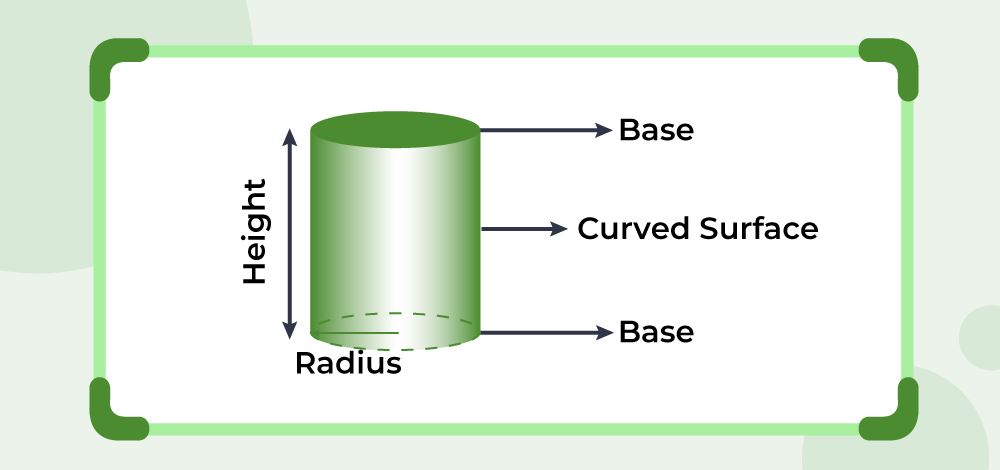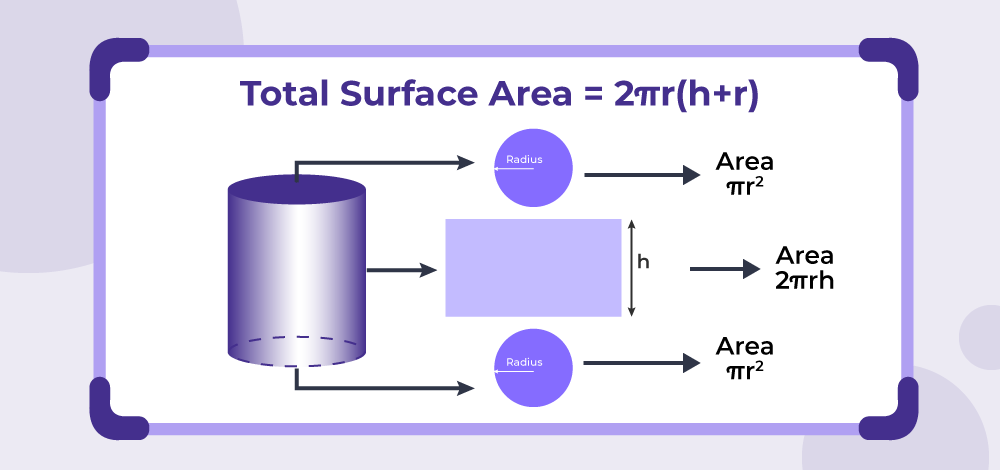Open in App
Not now

# Surface Area of a Cylinder

• Difficulty Level : Easy
• Last Updated : 19 Mar, 2023

Surface area of a cylinder is defined as the amount of space covered by the flat surface of the cylinder’s bases and the curved surface of the cylinder. The overall surface area of the cylinder includes the area of the cylinder’s two circular bases as well as the area of the curving surface. For a detailed explanation and derivation of the surface area of the cylinder formula along with examples read the article below

## What is the Surface Area of the Cylinder?

A cylinder is a three-dimensional structure formed by two parallel circular bases connected by a curving surface. The circular bases’ centres overlap each other to form a right cylinder. The axis is the line segment that connects the two centres and represents the height of the cylinder. The top view of the cylinder resembles a circle, while the side view resembles a rectangle. A cylinder, unlike a cone, cube, or cuboid, lacks vertices due to its curved form and lack of straight lines. It features two concentric circles on its face.

The following image shows the Surface Area of the Cylinder## Surface Area of Cylinder Formula

The cylinder’s surface area formula is used to calculate the surface area occupied by the cylinder’s bases and curving surface. Because a cylinder has a curved surface, we may express both its curved surface area and overall surface area. A cylinder has two types of surface areas: total surface area and curved surface area. We will discuss both of them one by one.

## Curved Surface Area of a Cylinder

The curved surface area of the cylinder is enclosed between the two parallel circular bases. It is also known as the lateral surface area. The formula is as follows:

Curved Surface Area = 2πrh

where,
r is the radius of the cylinder
h is the height of cylinder

Example: Calculate the curved surface area of the cylinder of radius 7 cm and height of 44 cm.

Solution:

We have, r = 7 cm and h = 44 cm

Curved surface area of cylinder = 2πrh

= 2 (22/7) (7) (44)

= 2 (22) (5) (2)

= 1936 cm2

## Total Surface Area of a Cylinder

A cylinder’s total surface area is the sum of its curved surface area and the area of its two circular bases. It is calculated by summing the areas of the two bases and the curved surface. As a result, the formula for the cylinder’s total surface area is as follows:

Total Surface Area of Cylinder  = 2πr2 + 2πrh = 2πr(r + h)

where,
r is the radius of the cylinder
h is the height of cylinder

Example: Calculate the total surface area of the cylinder of radius 7 cm and height of 10 cm.

Solution:

We have, r = 7 and h = 10.

Total surface area = 2πr2 + 2πrh

= 2 (22/7) (7)2 + 2 (22/7) (7) (10)

= 2 (22)(7) + 2 (22) (10)

= 308 + 440

= 748 sq. cm

## Derivation of Surface Area of Cylinder Formula

Consider a cylinder whose radius is r and height is h.

The cylinder is divided into three parts: one circular base at top, one rectangular curved area and another circular base at bottom as in the following image.The rectangular area has length of 2πr and breadth of h. So, the area is, A1 = 2πrh, which is also the curved surface area of the cylinder.

The area of a circular base with radius r is given by, πr2. So, the area of two such bases is, A2 = (πr2 + πr2) = 2πr2.

Now, the total surface area of the cylinder is the sum of above two areas.

A = A1 + A2

= 2πr2 + 2πrh

= 2πr(r + h)

This derives the formula for surface area of a cylinder.

## How to Find the Surface Area of a Cylinder?

Surface area of a cylinder is the area occupied by the bases of cylinder and the area of curved surface of cylinder. Use the steps given below, to find the total surface area of a cylinder that has a radius of 14 cm and a height of 10 cm.

Step 1: Mark the radius, ‘r’, and height, ‘h’ of cylinder. Remember both have the same units. Here, given r = 14 cm, h = 10 cm

Step 2: Here, we have to find the total surface area of the cylinder, the formula for the total surface area of the cylinder = 2πr(r + h)

Step 3: Put the given values in the above formulas and find the answer in square units. Substitute the values in the formula we get,

Total Surface Area = 2πr(r + h)
= 2π × 14(14 + 10)
= 2π × 336
= 2 × 3.14 × 336
Total Surface Area = 2110.08 square cm

## Volume of Cylinder

The volume of cylinder is defined as the total amount of space occupied by the cylinder. It is also defined as the total amount of liquid a cylinder can hold.

For a cylinder of base radius r, and height h the volume is calculated using the formula,

Volume of Cylinder = πr2h

For more detail check, Volume of a Cylinder

## Differences between TSA and CSA of Cylinder

Total Surface Area (TSA) of the cylinder and the Curved Surface Area (CSA) of the Cylinder are the two concepts that are highly used to calculate various things regarding the cylinder. The main difference between them is that CSA as the name suggest is the area of the curved surface of the cylinder whereas the TSA is the total area of the cylinder which includes CSA and the area of the two circular bases. The main differences between them can be learnt with help of the table given below.

## Solved Examples on Surface Area of Cylinder

Example 1: Find the curved surface area of the cylinder of radius 3 cm and height of 7 cm.

Solution:

We have, r = 3 and h = 7.

Curved surface area of cylinder = 2πrh

= 2 (22/7) (3) (7)

= 2 (22) (3)

= 132 cm2

Example 2: Find the radius of the cylinder of curved surface area of 220 sq. cm and height of 7 cm.

Solution:

We have, A = 220 and h = 7.

Curved surface area of cylinder = 2πrh

220 = 2 (22/7) (r) (7)

220 = 44r

r = 220/44

r = 5 cm

Example 3: Find the total surface area of the cylinder of radius 21 cm and height of 42 cm.

Solution:

We have, r = 21 and h = 42.

Total surface area = 2πr2 + 2πrh

= 2 (22/7) (21) (21) + 2 (22/7) (21) (42)

= 2 (22) (3) (21) + 2 (22) (3) (42)

= 2772 + 5544

= 8316 sq. cm

Example 4: Find the total surface of the cylinder if the curved surface area is 176 sq. cm and the height is 21 cm.

Solution:

We have, A = 176 and h = 21.

Curved surface area of cylinder = 2πrh

176 = 2 (22/7) (r) (21)

176 = 2 (22) (r) (3)

r = 176/132

r = 1.33 cm

Total surface area = 2πr2 + 2πrh

= 2 (3.14) (1.33) (1.33) + 176

= 11.10 + 176

= 187.1 sq. cm

Example 5: Find the radius of a cylinder if the sum of its height and radius is 7 cm such that the total surface area is 440 sq. cm.

Solution:

We have, (r + h) = 7 and A = 440.

Total surface area = 2πr(r + h)

440 = 2 (22/7) (r) (7)

2 (22) (r) = 440

44r = 440

r = 10 cm

Example 6: Find the curved surface area of a cylinder if its total surface area is 528 sq. cm and its radius is 7 cm.

Solution:

We have, A = 528 and r = 7 cm.

Total surface area = 2πr(r + h)

528 = 2 (22/7) (7) (7 + h)

2 (22) (7 + h) = 528

7 + h = 12

h = 5 cm

Curved surface area of cylinder = 2πrh

= 2 (22/7) (7) (5)

= 2 (22) (5)

= 220 sq. cm

## FAQs on Surface Area of Cylinder

### Question 1: What is the cylinder?

A cylinder is a three-dimensional shape having two circular bases in parallel to each other joined by a curved surface.

### Question 2: How to find the surface area of a cylinder?

For finding the surface area of a cylinder, we will find the surface area of curved surface and area of the circular bases of the cylinder. Now add all the areas to get the total surface area.

### Question 3: Write the formula for finding the area of the cylinder.

Formula for finding the area of a cylinder is,

• Total Surface Area = 2πr (h + r) sq. unit
• Curved Surface Area = 2πrh sq. unit

where,
h is the height of the cylinder

### Question 4: Write the formula for the volume of a cylinder.

Formula for finding the volume of a cylinder is,

Volume of cylinder (V) = πr2h cubic units.

where,
r is the radius of the base of cylinder
h is the height of the cylinder

### Question 5: How to Find the Surface Area of an Open Top Cylinder?

Surface area of an open-top cylinder can be calculated by finding the area of bottom circular base and the curved surface of the cylinder and then adding both the result. Thus,

Surface Area of an Open-Top Cylinder = πr(r + 2h)

where,
‘h’ is the height of the cylinder

### Question 6: What is the surface area for cylinder?

The 2-D space occupied by the surface area of the cylinder is called the surface area of the cylinder it is defined as the area of both bases of the cylinder along with its curved surface area.

### Question 7: What is the outer surface area of a hollow cylinder?

For a hollow cylinder with an outer radius R and inner radius r the outer surface area is defined as the curved area of the outer surface of the cylinder. It can be calculated using the formula,

Outer Surface Area = 2πRh

where,
R is outer radius of cylinder
h is height of cylinder

### Question 8: What is inner curved surface area of a hollow cylinder?

For a hollow cylinder with an outer radius R and inner radius r the inner surface area is defined as the curved area of the inner surface of the cylinder. It can be calculated using the formula,

Inner Surface Area = 2πrh

where,
r is inner radius of cylinder
h is height of cylinder

My Personal Notes arrow_drop_up
Related Articles